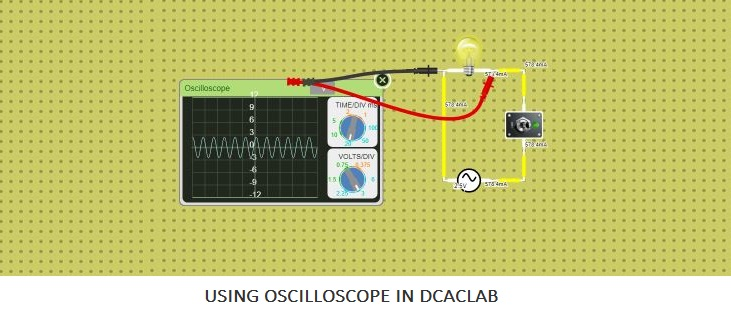# How to use Oscilloscope in Lab

Follow the below simple steps in order to use the oscilloscope in dcaclab.

Step 1: Navigate through the list of the devices/components as shown in image.Step 2: Click on the oscilloscope icon, the oscilloscope will then appear in the board below.Step 3: Grab the probes to measure the voltage of any point or define the signal at any point of the circuit.## How to use Time and Voltage Division in an OscillatorIn the above image shown of an oscilloscope there are two pivots, upper pivot is used to vary the time per division in the x axis and lower pivot is used to vary the voltage per division in the y axis.

You can play with the above circuit in here.

## What Can Oscilloscope Measure

With the Oscilloscope you can define the quantities like amplitude, frequency and other waveform characteristics in a Signal or AC current.

In easy way,

With the help of oscilloscope we can measure both the characteristics based on time as well as voltage.

### Timing characteristics

The time unit is shown by the x axis in the oscilloscope, with the help of time unit we can define the characteristics such as Frequency and period, Duty cycle and Rise and fall time.

#### Frequency and period

The frequency of the signal or a current (AC Current) is defined as the number of cycle the waveform completes in one second. The period of the current (AC Current) or a signal is defined as the opposite to the frequency that is the number of second each wave cycle takes.

#### Duty cycle

The duty cycle is calculated as the percentage of the signal weather it is positive or negative, The duty cycle is defined in two types, they are positive duty cycle and negative duty cycle.

With the help of Duty cycle we can define the ratio of the time period the signal is positive and the signal is negative.

#### Rise and fall time

The AC voltage or a signal does not instantaneously go 0v to 12v, they rise to the peak voltage instead. The Rise Time is defined as the time duration in which the signal goes from low point to high point, The Fall Time is defines the opposite that is the time duration in which the signal goes from high point to low point.

### Voltage characteristics

The voltage unit is shown by the y axis in the oscilloscope. With the help of Voltage unit we can define the characteristics such as Amplitude, Maximum and minimum voltages and Mean and average voltages.

#### Amplitude

The amplitude is defined as the magnitude of the voltage or a signal. There are many ways to define the amplitude, in one method the difference between high voltage point to low voltage point also known as peak-to-peak amplitude. In another method the distance from high voltage point or low voltage point to 0V.

#### Maximum and minimum voltages

With the help of Oscilloscope you can very easily define that how high and low does the voltage of your signal gets.

#### Mean and average voltages

With the help of oscilloscope you can very easily calculate the average or mean voltage of the signal. Also with the help of the oscilloscope you can define the average of the signal’s maximum as well as minimum voltage.

Do write on the comment below that how did you used the oscilloscope.

Click to see the use of oscilloscope in dcaclab.

•
3
Shares
• 3
•
•
•
•